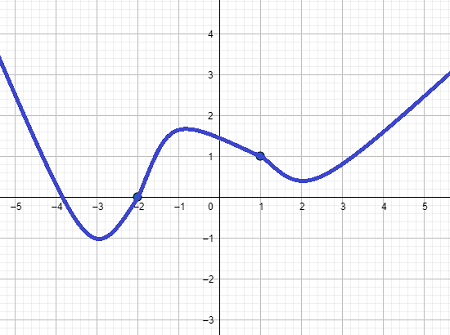# Sketch a graph of a single function that has these properties. a) Continuous and differentiable...

## Question:

Sketch a graph of a single function that has these properties.

a) {eq}\;\;\textrm{Continuous and differentiable for all real numbers} {/eq}

b) {eq}\;\;f'(x) > 0\; {/eq} on {eq}(-3,-1) {/eq} and {eq}(2,\infty) {/eq}

c) {eq}\;\;f'(x) < 0\; {/eq} on {eq}(-\infty,-3) {/eq} and {eq}(-1,2) {/eq}

d) {eq}\;\;f''(x) > 0\; {/eq} on {eq}(-\infty,-2) {/eq} and {eq}(1,\infty) {/eq}

e) {eq}\;\;f''(x) < 0\; {/eq} on {eq}(-2,1) {/eq}

f) {eq}\;\;f'(-3) = f'(-1) = f'(2) = 0 {/eq}

g) {eq}\;\;f''(x) = 0\; {/eq} at {eq}(-2,0) {/eq} and {eq}(1,1) {/eq}

## Concavity of Graphs

The graph of a function {eq}f(x) {/eq} is concave upward if the curve bends like one side of a smile. A concave upward function satisfies {eq}f''(x)>0. {/eq} The graph of the function is concave downward if the curve of the graph bends like one side of a frown. A concave downward function satisfies {eq}f''(x)<0. {/eq} Any point at which the function changes from concave upward to concave downward, or vice versa, is called an inflection point.

a) {eq}\;\;\textrm{Continuous and differentiable for all real numbers} {/eq}

This property means that there are no holes, vertical asymptotes, jumps, or sharp corners in the curve.

b) {eq}\;\;f'(x) > 0\; {/eq} on {eq}(-3,-1) {/eq} and {eq}(2,\infty) {/eq}

This means the function is increasing on {eq}(-3,-1)\cup(2,\infty) {/eq}

c) {eq}\;\;f'(x) < 0\; {/eq} on {eq}(-\infty,-3) {/eq} and {eq}(-1,2) {/eq}

This means the function is decreasing on {eq}(-\infty, -3)\cup(-1,2) {/eq}

d) {eq}\;\;f''(x) > 0\; {/eq} on {eq}(-\infty,-2) {/eq} and {eq}(1,\infty) {/eq}

This means the function is concave upward on {eq}(-\infty,-2)\cup(1,\infty) {/eq}

e) {eq}\;\;f''(x) < 0\; {/eq} on {eq}(-2,1) {/eq}

This means the function is concave downward on {eq}(-2,1) {/eq}

f) {eq}\;\;f'(-3) = f'(-1) = f'(2) = 0 {/eq}

This means the slope of the tangent line at {eq}x=-3,-1,2 {/eq} is zero - we have local maxima or minima at these values.

g) {eq}\;\;f''(x) = 0\; {/eq} at {eq}(-2,0) {/eq} and {eq}(1,1) {/eq}

This means our graph has inflection points at {eq}(-2,0) {/eq} and {eq}(1,1) {/eq}

Putting it all together, we have a sketch of the function below.